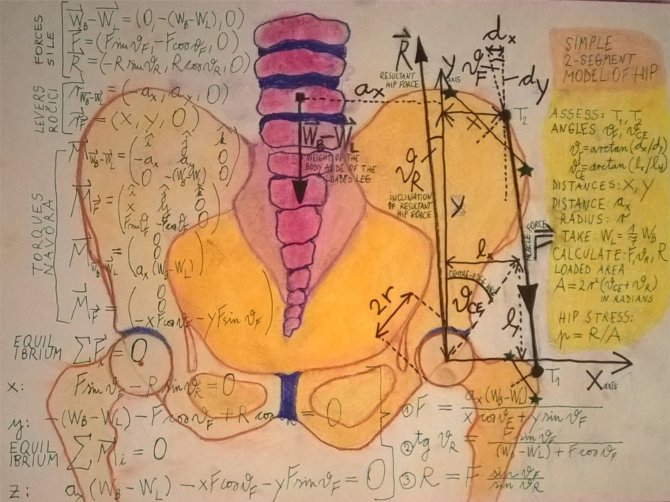Simple model of resultant hip force and hip stress in the one-legged stance

On the left side, forces and lever arms are defined and the equilibrium equations are given to formulate the problem. There are three unknowns, i.e. the magnitude and the inclination of the resultant hip force R, and the magnitude of the effective muscle force F.

On the right side, instructions are given to calculate the resultant hip force and the hip stress.On the left side, forces and lever arms are defined and the equilibrium equations are given to formulate the problem. There are three unknowns, i.e. the magnitude and the inclination of the resultant hip force R, and the magnitude of the effective muscle force F.

On the right side, instructions are given to calculate the resultant hip force and the hip stress.